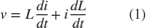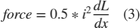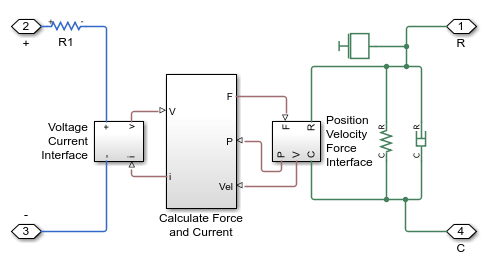# Solenoid

This example shows a solenoid with a spring return. The solenoid is modeled as an inductance whose value L depends on the plunger position x. The back emf for a time-varying inductance is given by:As L depends on x, this becomes:can be derived from manufacturer force-stroke data using the relationship:can then be integrated to get L as a function of x.

In the model, equation 2 is rearranged to solve for i, and then implemented using Physical Signal blocks. A controlled current source then constrains the current to equate to i.

### Model### Solenoid Subsystem### Simulation Results from Simscape Logging